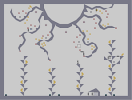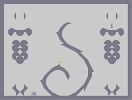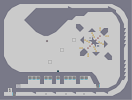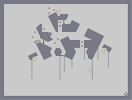### Blair-ChanHover over the thumbnail for a full-size version.

Author darkcrusader author:darkcrusader manga mines n-art non-playable rated soul-eater 2010-04-07 2011-08-26 3 by 50 people. \$Blair-Chan#darkcrusader#non-playable#00000000000000000000000000000000000000000000000000000000000000000000000000000000000000000000000000000000000000000000000000000000000000000000000000000000000000000000000000000000000000000000000000000000000000000000000000000000000000000000000000000000000000000000000000000000000000000000000000000000000000000000000000000000000000000000000000000000000000000000000000000000000000000000000000000000000000000000000000000000000000000000000000000000000000000000000000000000000000000000000000000000000000000000000000000000000000000000000000000000000000000000000000000000000000000000000000000000000000000000000000000000000000000000000000000000000000000000000000000000000000000000000000000000000000000000000000000000000000000|12^135,27!12^131,29!12^125,31!12^119,34!12^115,36!12^110,38!12^113,96!12^111,131!12^108,40!12^113,90!12^113,83!12^113,99!12^113,104!12^113,111!12^112,114!12^112,121!12^111,126!12^107,163!12^107,158!12^108,153!12^108,147!12^110,143!12^110,139!12^110,136!12^106,168!12^167,28!12^162,31!12^157,33!12^153,34!12^150,36!12^147,38!12^141,42!12^136,45!12^136,48!12^136,58!12^136,64!12^136,75!12^136,68!12^136,52!12^136,83!12^135,85!12^135,91!12^135,98!12^135,106!12^134,113!12^134,118!12^133,126!12^132,134!12^131,140!12^112,77!12^112,73!12^111,68!12^111,64!12^111,60!12^109,56!12^108,53!12^108,49!12^105,44!12^168,156!12^135,163!12^106,171!12^113,169!12^119,167!12^125,165!12^129,164!12^143,161!12^146,160!12^153,159!12^158,158!12^164,157!12^184,154!12^178,154!12^171,155!12^201,152!12^195,152!12^192,152!12^187,152!12^208,152!12^213,152!12^216,150!12^216,144!12^216,138!12^216,132!12^216,126!12^216,120!12^216,114!12^216,108!12^216,96!12^216,102!12^216,90!12^216,84!12^216,78!12^216,72!12^133,140!12^140,138!12^146,136!12^151,136!12^159,134!12^165,134!12^172,133!12^178,133!12^185,132!12^192,131!12^156,135!12^198,132!12^198,126!12^198,114!12^198,108!12^198,102!12^198,120!12^198,96!12^155,84!12^164,83!12^159,83!12^171,81!12^178,80!12^183,79!12^188,77!12^193,77!12^198,75!12^205,74!12^213,72!12^194,93!12^188,92!12^181,92!12^175,92!12^167,93!12^165,95!12^165,101!12^165,106!12^165,110!12^153,87!12^153,91!12^153,96!12^153,100!12^153,107!12^153,113!12^153,119!12^158,123!12^154,124!12^166,122!12^165,122!12^172,121!12^180,119!12^176,119!12^180,114!12^180,109!12^179,105!12^176,105!12^175,108!12^175,111!12^171,115!12^168,115!12^165,116!12^239,29!12^230,34!12^224,37!12^236,31!12^225,39!12^246,26!12^242,29!12^337,53!12^265,70!12^301,63!12^281,47!12^306,50!12^316,58!12^281,68!12^251,44!12^293,49!12^265,45!12^238,43!12^224,40!12^233,41!12^230,41!12^248,44!12^242,44!12^261,45!12^254,45!12^276,46!12^269,46!12^287,48!12^303,50!12^299,50!12^314,52!12^330,54!12^324,53!12^318,53!12^311,52!12^319,59!12^311,61!12^305,62!12^294,65!12^286,67!12^282,67!12^274,68!12^267,71!12^260,71!12^252,73!12^253,118!12^249,77!12^249,81!12^250,88!12^252,94!12^252,98!12^253,107!12^253,114!12^253,103!12^254,125!12^254,132!12^253,140!12^252,146!12^252,152!12^251,158!12^250,165!12^250,172!12^248,179!12^245,184!12^363,168!12^357,233!12^232,207!12^234,203!12^238,197!12^240,189!12^244,185!12^250,184!12^231,213!12^222,230!12^216,234!12^232,217!12^228,223!12^223,228!12^231,221!12^225,227!12^353,234!12^349,232!12^343,231!12^337,231!12^329,231!12^319,231!12^325,231!12^314,232!12^310,234!12^304,234!12^298,235!12^294,236!12^287,237!12^282,237!12^278,238!12^270,239!12^263,239!12^256,239!12^272,239!12^259,239!12^249,239!12^241,239!12^237,238!12^234,236!12^230,236!12^223,236!12^217,236!12^257,182!12^262,180!12^267,179!12^272,178!12^280,176!12^285,176!12^364,165!12^364,158!12^359,158!12^352,158!12^343,160!12^335,161!12^327,164!12^321,165!12^302,170!12^288,174!12^315,166!12^307,168!12^293,173!12^297,171!12^279,177!12^311,166!12^331,163!12^346,159!12^362,172!12^362,180!12^361,186!12^361,192!12^360,197!12^360,203!12^360,208!12^361,209!12^361,217!12^363,218!12^362,224!12^362,231!12^362,234!12^354,178!12^353,174!12^348,172!12^344,172!12^339,180!12^339,184!12^341,189!12^346,191!12^348,190!12^352,186!12^353,181!12^340,217!12^341,213!12^346,209!12^352,210!12^354,213!12^354,219!12^340,219!12^343,224!12^351,223!12^428,42!12^422,43!12^417,44!12^413,46!12^407,48!12^400,49!12^395,51!12^385,52!12^381,53!12^375,55!12^371,56!12^366,59!12^391,50!12^434,39!12^428,37!12^400,31!12^396,29!12^390,27!12^385,27!12^364,60!12^430,76!12^397,68!12^412,72!12^422,37!12^417,35!12^412,34!12^406,31!12^380,64!12^424,75!12^417,73!12^407,70!12^402,68!12^392,67!12^388,66!12^386,65!12^378,64!12^371,61!12^429,172!12^424,123!12^427,96!12^427,151!12^428,166!12^427,161!12^427,157!12^425,148!12^425,139!12^424,137!12^424,132!12^424,129!12^425,118!12^425,112!12^426,110!12^426,108!12^426,105!12^427,104!12^427,99!12^428,93!12^428,91!12^429,89!12^429,85!12^429,83!12^430,81!12^431,78!12^424,141!12^367,169!12^442,181!12^438,179!12^434,178!12^430,177!12^425,177!12^420,174!12^417,173!12^404,169!12^397,168!12^392,168!12^385,168!12^379,168!12^374,168!12^371,171!12^410,170!12^406,169!12^448,182!12^448,186!12^448,191!12^448,195!12^448,199!12^448,203!12^448,206!12^448,211!12^448,217!12^448,222!12^448,227!12^448,232!12^448,234!12^443,235!12^438,235!12^435,234!12^429,233!12^425,233!12^420,233!12^419,232!12^414,232!12^409,232!12^406,231!12^401,231!12^395,231!12^392,231!12^391,230!12^387,230!12^383,230!12^380,230!12^378,230!12^371,231!12^369,231!12^367,231!12^408,212!12^414,215!12^418,218!12^423,221!12^259,202!12^263,205!12^267,209!12^269,213!12^257,200!12^254,198!12^266,215!12^263,217!12^400,206!12^404,209!12^342,174!12^340,176!12^323,154!12^320,150!12^314,144!12^310,140!12^305,135!12^301,129!12^295,123!12^290,117!12^288,114!12^328,157!12^341,156!12^335,148!12^331,142!12^326,137!12^323,131!12^316,123!12^313,117!12^309,113!12^304,107!12^301,102!12^301,98!12^301,93!12^303,89!12^372,52!12^379,48!12^383,45!12^386,42!12^389,39!12^391,38!12^384,36!12^381,34!12^376,29!12^322,128!12^326,134!12^332,139!12^337,145!12^340,151!12^287,117!12^287,123!12^288,128!12^286,133!12^287,142!12^287,147!12^286,138!12^285,149!12^285,154!12^285,160!12^285,165!12^310,85!12^316,82!12^338,70!12^322,79!12^329,76!12^335,72!12^343,67!12^347,64!12^353,62!12^358,59!12^363,57!12^368,51!12^374,50!12^366,55!12^286,171!12^141,270!12^211,237!12^176,253!12^193,246!12^159,262!12^202,242!12^184,250!12^166,258!12^150,266!12^146,268!12^155,264!12^164,261!12^169,258!12^173,255!12^180,253!12^191,247!12^198,244!12^206,240!12^138,270!12^132,270!12^126,270!12^120,270!12^114,270!12^102,270!12^108,270!12^96,270!12^90,270!12^84,270!12^78,270!12^72,270!12^66,270!12^60,270!12^54,270!12^48,270!12^42,270!12^36,270!12^30,270!12^48,287!12^42,283!12^38,280!12^34,274!12^64,292!12^60,291!12^55,289!12^49,286!12^85,297!12^92,299!12^77,295!12^69,293!12^97,300!12^83,297!12^76,295!12^171,310!12^165,309!12^162,308!12^157,308!12^154,308!12^150,308!12^149,306!12^144,306!12^138,306!12^136,306!12^133,306!12^132,305!12^129,305!12^126,305!12^122,305!12^121,304!12^119,304!12^116,304!12^114,303!12^111,303!12^106,303!12^106,302!12^103,302!12^100,302!12^358,257!12^177,311!12^182,311!12^185,311!12^192,310!12^197,308!12^205,305!12^201,307!12^210,303!12^217,300!12^223,296!12^229,293!12^235,291!12^239,289!12^245,286!12^252,284!12^258,282!12^264,278!12^269,276!12^275,273!12^282,272!12^290,269!12^300,267!12^296,267!12^308,264!12^316,263!12^321,262!12^329,261!12^337,259!12^343,256!12^352,256!12^356,256!12^329,246!12^324,244!12^320,242!12^314,240!12^308,240!12^303,238!12^325,247!12^320,248!12^313,250!12^308,253!12^302,254!12^297,256!12^292,259!12^286,262!12^280,265!12^274,267!12^268,269!12^262,270!12^253,272!12^246,275!12^239,277!12^231,280!12^257,269!12^264,269!12^270,267!12^275,265!12^280,262!12^268,265!12^263,267!12^228,282!12^224,285!12^219,287!12^212,290!12^207,293!12^200,295!12^194,297!12^190,299!12^186,301!12^182,303!12^172,304!12^222,300!12^222,306!12^222,312!12^222,318!12^222,324!12^222,330!12^516,263!12^511,258!12^505,255!12^500,254!12^496,252!12^489,248!12^485,247!12^480,245!12^477,244!12^473,243!12^465,240!12^461,238!12^454,238!12^468,242!12^448,234!12^491,248!12^513,259!12^446,266!12^440,265!12^436,264!12^432,263!12^425,262!12^419,260!12^412,259!12^405,258!12^397,257!12^391,256!12^383,256!12^377,255!12^372,255!12^365,255!12^355,255!12^346,256!12^339,257!12^332,257!12^321,259!12^325,259!12^450,265!12^454,265!12^458,267!12^463,269!12^468,271!12^474,271!12^480,273!12^488,275!12^494,275!12^500,277!12^507,280!12^514,283!12^520,286!12^528,289!12^522,264!12^528,264!12^534,264!12^540,264!12^546,264!12^558,264!12^564,264!12^570,264!12^552,264!12^582,264!12^576,264!12^588,264!12^600,264!12^594,264!12^606,264!12^612,264!12^618,264!12^534,291!12^542,292!12^549,292!12^556,292!12^562,291!12^566,290!12^606,285!12^616,282!12^572,290!12^580,290!12^587,290!12^594,289!12^599,288!12^604,286!12^610,285!12^630,276!12^636,272!12^626,278!12^621,280!12^641,271!12^647,267!12^624,264!12^630,264!12^636,264!12^642,264!12^648,264!12^654,264!12^223,336!12^224,341!12^291,401!12^290,402!12^228,351!12^226,346!12^229,355!12^232,358!12^232,358!12^235,362!12^238,367!12^242,373!12^248,377!12^254,383!12^269,392!12^264,390!12^261,388!12^286,401!12^281,399!12^276,397!12^441,269!12^449,319!12^449,315!12^449,309!12^449,304!12^448,301!12^447,296!12^447,291!12^447,289!12^445,288!12^445,284!12^445,278!12^444,277!12^443,274!12^415,376!12^422,370!12^427,365!12^434,358!12^438,353!12^441,347!12^445,341!12^388,393!12^385,395!12^379,399!12^370,402!12^358,405!12^354,405!12^348,408!12^343,409!12^337,409!12^333,409!12^328,409!12^324,408!12^320,408!12^316,408!12^314,407!12^312,407!12^310,407!12^308,406!12^306,406!12^304,406!12^301,404!12^297,404!12^294,403!12^352,407!12^373,401!12^367,403!12^361,405!12^394,391!12^398,388!12^403,385!12^408,382!12^411,379!12^431,361!12^419,373!12^425,367!12^258,386!12^253,380!12^309,412!12^304,417!12^301,425!12^299,430!12^298,439!12^298,435!12^302,420!12^306,415!12^299,443!12^299,450!12^300,454!12^304,463!12^302,460!12^309,467!12^313,467!12^317,464!12^325,459!12^321,463!12^326,457!12^329,454!12^329,447!12^330,441!12^330,437!12^330,429!12^329,427!12^329,422!12^326,419!12^325,414!12^427,370!12^429,375!12^447,335!12^448,329!12^448,325!12^470,417!12^466,415!12^461,412!12^454,408!12^448,402!12^444,398!12^439,395!12^434,390!12^432,386!12^430,381!12^451,405!12^458,410!12^478,420!12^505,430!12^501,427!12^496,425!12^491,423!12^483,421!12^489,423!12^530,444!12^517,436!12^525,441!12^521,438!12^516,435!12^513,433!12^509,431!12^535,448!12^312,472!12^314,478!12^314,484!12^315,490!12^317,498!12^323,509!12^324,522!12^324,516!12^322,505!12^326,531!12^326,529!12^328,537!12^330,543!12^326,548!12^320,552!12^315,555!12^308,558!12^305,560!12^301,566!12^300,572!12^334,559!12^337,565!12^340,569!12^340,573!12^412,388!12^408,396!12^404,404!12^400,410!12^396,417!12^392,425!12^389,430!12^384,438!12^380,444!12^375,451!12^372,457!12^369,460!12^364,467!12^362,472!12^358,476!12^356,483!12^353,495!12^351,502!12^423,440!12^428,448!12^431,455!12^433,464!12^434,475!12^434,483!12^433,490!12^356,488!12^353,504!12^352,510!12^352,521!12^353,515!12^354,528!12^358,534!12^359,543!12^359,551!12^363,575!12^362,568!12^362,562!12^362,559!12^361,558!12^361,551!12^360,548!12^360,544!12^360,541!12^432,497!12^432,502!12^428,519!12^424,526!12^418,536!12^416,542!12^421,531!12^414,548!12^412,555!12^411,562!12^410,567!12^410,572!12^410,574!12^482,504!12^477,504!12^472,504!12^464,506!12^459,506!12^453,508!12^446,511!12^440,512!12^438,514!12^434,517!12^430,521!12^538,450!12^542,452!12^547,457!12^550,460!12^555,466!12^560,471!12^564,475!12^569,480!12^573,485!12^576,490!12^579,497!12^582,503!12^585,510!12^587,517!12^589,528!12^588,524!12^591,536!12^596,543!12^593,540!12^602,548!12^609,549!12^617,549!12^625,548!12^632,548!12^644,547!12^637,547!12^650,546!12^660,541!12^667,538!12^675,535!12^685,531!12^680,533!12^655,545!12^594,540!12^594,547!12^594,552!12^689,530!12^695,527!12^700,525!12^706,523!12^712,520!12^717,518!12^723,514!12^730,509!12^735,505!12^740,500!12^746,496!12^752,489!12^758,483!12^763,478!12^767,471!12^753,492!12^673,536!12^662,544!12^767,530!12^763,535!12^758,540!12^753,543!12^747,547!12^742,551!12^736,554!12^731,556!12^719,565!12^716,568!12^712,569!12^708,572!12^723,562!12^729,558!12^704,574!12^698,575!12^691,576!12^682,576!12^685,576!12^612,549!12^627,549!12^412,267!12^404,267!12^399,267!12^418,269!12^391,267!12^387,267!12^383,266!12^378,266!12^376,266!12^387,319!12^392,319!12^396,318!12^401,316!12^420,273!12^422,279!12^421,286!12^420,292!12^418,297!12^416,303!12^413,308!12^408,312!12^406,314!12^385,319!12^378,319!12^373,315!12^369,313!12^365,308!12^362,305!12^359,298!12^358,292!12^356,285!12^355,281!12^365,268!12^356,275!12^359,272!12^385,288!12^383,285!12^380,282!12^379,277!12^378,273!12^386,285!12^387,283!12^388,277!12^388,273!12^404,323!12^410,320!12^418,319!12^414,318!12^424,316!12^422,317!12^431,314!12^435,315!12^399,326!12^396,329!12^396,334!12^421,340!12^428,339!12^431,337!12^436,333!12^441,329!12^443,324!12^443,322!12^440,319!12^338,385!12^359,357!12^357,362!12^351,375!12^354,369!12^347,379!12^341,384!12^334,385!12^328,384!12^324,382!12^320,377!12^317,371!12^315,367!12^313,363!12^313,358!12^335,351!12^330,355!12^327,357!12^320,357!12^314,358!12^355,358!12^351,358!12^347,357!12^343,355!12^339,352!12^335,335!12^339,331!12^335,321!12^340,325!12^329,322!12^324,324!12^325,330!12^328,333!12^243,295!12^241,291!12^244,301!12^254,315!12^251,311!12^247,304!12^249,309!12^303,271!12^303,277!12^303,284!12^302,288!12^301,293!12^300,301!12^297,306!12^294,314!12^288,317!12^285,319!12^280,321!12^274,322!12^269,322!12^265,321!12^260,319!12^296,311!12^301,296!12^290,293!12^287,288!12^285,284!12^284,280!12^284,277!12^292,289!12^293,285!12^294,280!12^294,274!12^264,332!12^257,329!12^252,329!12^247,329!12^241,329!12^237,328!12^231,328!12^266,333!12^270,336!12^270,341!12^266,343!12^264,345!12^260,345!12^255,345!12^248,345!12^242,344!12^237,343!12^233,343!12^229,340!12^226,336!12^229,334!12^126,138!12^126,132!12^126,126!12^126,120!12^132,114!12^132,108!12^132,102!12^132,96!12^132,84!12^132,90!12^132,72!12^132,78!12^132,66!12^132,60!12^132,48!12^132,54!12^132,42!12^138,36!12^144,36!12^150,30!12^162,24!12^126,144!12^210,150!12^210,144!12^210,138!12^210,132!12^210,126!12^210,120!12^210,114!12^210,108!12^210,102!12^210,96!12^210,90!12^210,84!12^210,78!12^128,116!12^129,108!12^129,101!12^130,94!12^130,88!12^130,83!12^131,82!12^131,77!12^131,75!12^131,71!12^288,168!12^294,168!12^288,162!12^294,162!12^300,162!12^312,162!12^306,162!12^318,162!12^324,162!12^318,156!12^312,156!12^306,156!12^300,156!12^294,156!12^294,156!12^288,156!12^288,150!12^294,150!12^300,150!12^306,150!12^318,150!12^312,150!12^306,144!12^300,144!12^294,144!12^300,138!12^294,138!12^294,132!12^294,126!12^420,168!12^420,168!12^426,168!12^414,168!12^408,168!12^402,168!12^366,162!12^372,162!12^378,162!12^384,162!12^390,162!12^396,162!12^402,162!12^408,162!12^420,162!12^426,162!12^414,162!12^420,156!12^414,156!12^408,156!12^402,156!12^396,156!12^390,156!12^384,156!12^378,156!12^372,156!12^366,156!12^360,156!12^354,156!12^348,156!12^420,150!12^420,144!12^420,138!12^420,132!12^420,126!12^420,120!12^420,114!12^420,108!12^420,102!12^420,96!12^420,90!12^420,84!12^420,78!12^426,78!12^426,84!12^426,90!12^414,78!12^408,78!12^402,78!12^396,78!12^390,78!12^384,78!12^408,72!12^402,72!12^396,72!12^390,72!12^384,72!12^378,72!12^378,78!12^372,78!12^372,72!12^366,72!12^366,78!12^360,78!12^360,72!12^354,72!12^354,78!12^348,78!12^348,72!12^348,66!12^360,66!12^372,66!12^378,66!12^366,66!12^354,66!12^342,72!12^342,78!12^336,78!12^330,78!12^324,84!12^330,84!12^318,84!12^312,84!12^336,84!12^342,84!12^348,84!12^354,84!12^360,84!12^366,84!12^372,84!12^384,84!12^378,84!12^390,84!12^396,84!12^402,84!12^408,84!12^414,84!12^414,90!12^414,96!12^414,102!12^414,108!12^414,120!12^414,114!12^414,126!12^414,132!12^414,138!12^414,150!12^414,144!12^408,144!12^408,150!12^402,150!12^402,144!12^396,138!12^390,138!12^390,132!12^396,132!12^390,144!12^390,150!12^396,150!12^396,144!12^402,138!12^408,138!12^408,132!12^402,132!12^390,126!12^396,126!12^402,126!12^408,126!12^408,120!12^402,120!12^396,120!12^390,120!12^384,120!12^384,126!12^384,132!12^384,138!12^384,144!12^384,150!12^378,150!12^378,144!12^378,138!12^378,132!12^378,132!12^378,126!12^378,120!12^372,120!12^372,126!12^372,132!12^372,138!12^372,144!12^372,150!12^366,150!12^366,138!12^366,144!12^366,132!12^366,126!12^366,120!12^360,120!12^360,126!12^360,132!12^360,138!12^360,144!12^360,150!12^354,150!12^354,144!12^354,132!12^354,138!12^354,126!12^354,126!12^354,120!12^348,150!12^348,144!12^348,138!12^348,132!12^348,126!12^348,120!12^342,120!12^342,126!12^342,132!12^342,138!12^342,144!12^342,150!12^336,138!12^336,132!12^336,126!12^336,120!12^330,120!12^330,126!12^330,132!12^324,126!12^324,120!12^324,120!12^318,120!12^318,114!12^324,114!12^330,114!12^336,114!12^342,114!12^348,114!12^360,114!12^354,114!12^366,114!12^372,114!12^378,114!12^384,114!12^390,114!12^396,114!12^402,114!12^408,114!12^408,108!12^402,108!12^396,108!12^390,108!12^384,108!12^372,108!12^378,108!12^366,108!12^366,108!12^360,108!12^354,108!12^348,108!12^342,108!12^336,108!12^330,108!12^324,108!12^324,108!12^318,108!12^312,108!12^306,102!12^312,102!12^312,96!12^306,96!12^306,90!12^312,90!12^318,90!12^324,90!12^336,90!12^330,90!12^342,90!12^348,90!12^354,90!12^360,90!12^372,90!12^366,90!12^378,90!12^384,90!12^396,90!12^402,90!12^408,90!12^390,90!12^408,96!12^408,102!12^402,102!12^402,96!12^396,96!12^396,102!12^384,102!12^390,96!12^384,96!12^378,96!12^378,102!12^366,102!12^372,96!12^372,102!12^390,102!12^366,96!12^360,96!12^360,102!12^354,102!12^354,96!12^348,96!12^348,102!12^342,102!12^342,96!12^336,96!12^336,102!12^330,102!12^330,96!12^324,96!12^324,102!12^318,102!12^318,90!12^318,90!12^318,96!12^330,150!12^324,144!12^318,138!12^312,132!12^306,126!12^312,120!12^300,120!12^306,114!12^294,114!12^300,108!12^288,108!12^294,102!12^282,102!12^288,96!12^294,90!12^282,90!12^276,96!12^276,108!12^282,114!12^276,120!12^282,126!12^276,132!12^282,138!12^276,144!12^282,150!12^276,156!12^282,162!12^276,168!12^270,162!12^270,150!12^270,138!12^270,126!12^270,114!12^270,102!12^264,168!12^270,174!12^258,174!12^258,162!12^264,156!12^258,150!12^264,144!12^258,138!12^264,132!12^258,126!12^264,120!12^258,114!12^264,108!12^258,102!12^264,96!12^258,90!12^270,90!12^252,84!12^264,84!12^276,84!12^288,84!12^300,84!12^258,78!12^270,78!12^282,78!12^294,78!12^306,78!12^276,72!12^288,72!12^300,72!12^312,72!12^324,72!12^306,66!12^318,66!12^330,66!12^324,60!12^336,60!12^342,54!12^348,60!12^354,54!12^348,48!12^360,48!12^372,48!12^378,42!12^366,42!12^354,42!12^360,36!12^372,36!12^366,30!12^354,30!12^360,24!12^372,24!12^378,24!12^384,30!12^390,36!12^396,36!12^402,36!12^390,30!12^408,36!12^414,36!12^414,42!12^408,42!12^402,42!12^396,42!12^390,42!12^396,48!12^390,48!12^384,48!12^348,36!12^348,24!12^342,30!12^342,42!12^336,48!12^336,36!12^336,24!12^330,30!12^330,42!12^324,48!12^324,36!12^324,24!12^318,30!12^318,42!12^312,48!12^312,36!12^312,24!12^306,30!12^378,36!12^372,30!12^366,24!12^306,42!12^294,42!12^300,36!12^294,30!12^300,24!12^288,24!12^288,36!12^282,42!12^282,30!12^276,24!12^276,36!12^264,36!12^270,42!12^270,30!12^264,24!12^258,30!12^258,42!12^252,36!12^252,24!12^246,30!12^240,36!12^138,30!12^144,24!12^156,24!12^132,36!12^120,36!12^126,42!12^114,42!12^120,48!12^114,54!12^126,54!12^120,60!12^126,66!12^114,66!12^120,72!12^126,78!12^114,78!12^120,84!12^126,90!12^120,96!12^126,102!12^120,108!12^126,114!12^120,120!12^120,132!12^120,144!12^114,126!12^114,138!12^114,150!12^126,150!12^120,156!12^108,156!12^114,162!12^126,162!12^132,156!12^138,150!12^144,156!12^150,150!12^144,144!12^132,144!12^156,144!12^162,150!12^156,156!12^168,144!12^174,150!12^180,144!12^186,150!12^192,144!12^198,150!12^186,138!12^174,138!12^162,138!12^150,138!12^198,138!12^204,144!12^204,132!12^204,120!12^204,108!12^204,96!12^204,84!12^198,90!12^198,78!12^192,84!12^180,84!12^168,84!12^174,90!12^186,90!12^162,90!12^156,96!12^162,102!12^156,108!12^162,114!12^156,120!12^444,558!12^444,552!12^444,546!12^444,540!12^444,534!12^450,534!12^456,534!12^462,540!12^456,546!12^462,552!12^456,558!12^450,558!12^450,546!12^474,534!12^474,540!12^474,546!12^474,552!12^474,558!12^480,558!12^486,558!12^498,558!12^498,552!12^498,546!12^498,540!12^504,534!12^510,534!12^516,540!12^516,546!12^516,552!12^516,558!12^510,546!12^504,546!12^528,558!12^534,558!12^540,558!12^534,552!12^534,546!12^534,540!12^534,534!12^528,534!12^540,534!12^552,558!12^552,552!12^552,546!12^552,540!12^552,534!12^558,534!12^564,534!12^570,540!12^564,546!12^558,546!12^570,552!12^570,558!12^318,234!12^324,234!12^336,234!12^330,234!12^342,234!12^348,234!12^360,234!12^366,234!12^372,234!12^378,234!12^384,234!12^390,234!12^396,234!12^402,234!12^414,234!12^408,234!12^420,234!12^426,234!12^432,234!12^444,234!12^450,234!12^456,240!12^450,240!12^444,240!12^438,240!12^432,240!12^426,240!12^420,240!12^414,240!12^408,240!12^402,240!12^396,240!12^390,240!12^384,240!12^372,240!12^378,240!12^366,240!12^360,240!12^354,240!12^348,240!12^342,240!12^336,240!12^330,240!12^324,240!12^462,240!12^330,246!12^336,246!12^342,246!12^348,246!12^354,246!12^360,246!12^366,246!12^372,246!12^378,246!12^384,246!12^390,246!12^396,246!12^402,246!12^408,246!12^414,246!12^420,246!12^426,246!12^432,246!12^438,246!12^444,246!12^456,246!12^450,246!12^462,246!12^468,246!12^474,246!12^480,246!12^378,252!12^372,252!12^366,252!12^360,252!12^354,252!12^348,252!12^342,252!12^336,252!12^330,252!12^324,252!12^318,252!12^312,252!12^312,258!12^306,258!12^318,258!12^300,258!12^300,264!12^294,264!12^288,264!12^312,264!12^300,264!12^306,264!12^300,264!12^294,264!12^288,264!12^282,264!12^258,276!12^252,276!12^246,282!12^240,282!12^234,282!12^252,276!12^252,282!12^246,276!12^228,288!12^234,288!12^222,288!12^222,294!12^216,294!12^210,294!12^210,300!12^204,300!12^198,300!12^198,306!12^192,306!12^186,306!12^390,252!12^396,252!12^384,252!12^402,252!12^408,252!12^420,252!12^414,252!12^426,252!12^432,252!12^438,252!12^444,252!12^450,252!12^456,252!12^462,252!12^468,252!12^474,252!12^480,252!12^486,252!12^492,252!12^426,258!12^432,258!12^438,258!12^444,258!12^450,258!12^456,258!12^468,258!12^462,258!12^348,216!12^348,180!12^438,228!12^444,222!12^438,216!12^444,210!12^438,204!12^444,198!12^438,192!12^444,186!12^432,186!12^432,198!12^432,210!12^432,222!12^462,264!12^468,264!12^474,264!12^474,258!12^480,258!12^480,264!12^486,270!12^486,264!12^486,258!12^492,258!12^498,258!12^504,258!12^492,264!12^498,264!12^504,264!12^510,264!12^516,264!12^480,270!12^474,270!12^462,264!12^456,264!12^444,264!12^420,258!12^414,258!12^408,258!12^402,258!12^492,270!12^498,270!12^504,270!12^510,270!12^522,270!12^516,270!12^528,270!12^534,270!12^546,270!12^540,270!12^552,270!12^564,270!12^558,270!12^570,270!12^582,270!12^576,270!12^588,270!12^594,270!12^600,270!12^606,270!12^612,270!12^618,270!12^618,270!12^624,270!12^630,270!12^636,270!12^642,270!12^624,276!12^618,276!12^612,276!12^606,276!12^600,276!12^594,276!12^582,276!12^588,276!12^576,276!12^570,276!12^564,276!12^558,276!12^552,276!12^546,276!12^540,276!12^534,276!12^528,276!12^522,276!12^516,276!12^510,276!12^426,204!12^504,276!12^522,282!12^528,282!12^534,282!12^540,282!12^546,282!12^552,282!12^558,282!12^564,282!12^576,282!12^570,282!12^582,282!12^588,282!12^594,282!12^606,282!12^600,282!12^612,282!12^576,288!12^570,288!12^582,288!12^588,288!12^594,288!12^564,288!12^558,288!12^552,288!12^552,288!12^546,288!12^534,288!12^540,288!12^606,552!12^606,558!12^606,564!12^606,570!12^600,558!12^600,564!12^600,570!12^612,558!12^618,558!12^624,558!12^612,552!12^618,552!12^624,552!12^630,552!12^630,558!12^636,558!12^636,552!12^642,558!12^648,558!12^648,552!12^642,552!12^654,552!12^654,558!12^660,558!12^660,552!12^660,546!12^666,540!12^666,546!12^666,546!12^666,552!12^666,558!12^672,558!12^672,552!12^672,546!12^672,540!12^672,564!12^666,564!12^660,564!12^660,564!12^654,564!12^648,564!12^642,564!12^636,564!12^630,564!12^624,564!12^618,564!12^612,564!12^606,576!12^594,576!12^600,576!12^612,570!12^612,576!12^594,570!12^594,570!12^594,564!12^594,564!12^594,558!12^618,570!12^618,576!12^624,576!12^624,570!12^630,570!12^630,570!12^630,576!12^636,576!12^636,570!12^642,570!12^642,576!12^648,576!12^648,570!12^654,570!12^654,576!12^660,576!12^660,570!12^666,570!12^666,576!12^672,576!12^672,570!12^678,570!12^678,576!12^678,564!12^684,564!12^684,570!12^690,570!12^690,564!12^696,564!12^696,570!12^702,570!12^702,564!12^708,564!12^714,564!12^678,558!12^678,552!12^684,546!12^678,540!12^678,546!12^684,534!12^684,540!12^684,552!12^684,558!12^696,558!12^690,558!12^690,552!12^696,552!12^714,558!12^714,552!12^720,552!12^720,558!12^720,540!12^714,540!12^714,534!12^714,528!12^714,522!12^708,522!12^702,522!12^696,528!12^690,534!12^696,534!12^708,528!12^708,534!12^708,540!12^726,552!12^726,546!12^726,540!12^720,546!12^714,546!12^708,546!12^708,552!12^708,558!12^702,558!12^702,552!12^702,546!12^696,546!12^690,546!12^690,540!12^696,540!12^702,540!12^702,534!12^702,528!12^726,534!12^720,534!12^720,528!12^726,528!12^726,522!12^720,522!12^726,516!12^732,522!12^732,528!12^732,534!12^732,540!12^732,546!12^732,552!12^738,546!12^738,540!12^738,534!12^738,528!12^738,522!12^732,516!12^738,516!12^738,510!12^738,504!12^744,504!12^744,510!12^744,516!12^744,522!12^744,528!12^744,528!12^744,540!12^744,546!12^744,534!12^750,534!12^750,540!12^750,528!12^750,522!12^750,516!12^750,510!12^750,504!12^750,498!12^756,492!12^756,498!12^756,504!12^756,510!12^756,522!12^726,558!12^720,516!12^732,510!12^708,570!12^738,552!12^744,498!12^756,528!12^756,534!12^756,516!12^762,516!12^762,522!12^762,528!12^762,510!12^762,504!12^762,498!12^762,492!12^762,486!12^768,522!12^768,516!12^768,510!12^768,504!12^768,498!12^768,492!12^768,486!12^768,480!12^768,474!12^756,486!12^762,480!12^426,192!12^420,210!12^426,216!12^426,228!12^414,228!12^420,222!12^414,204!12^420,198!12^414,192!12^420,186!12^426,180!12^414,180!12^408,222!12^402,228!12^402,216!12^396,222!12^390,228!12^384,222!12^378,228!12^372,222!12^366,228!12^366,216!12^372,210!12^366,204!12^372,198!12^366,192!12^372,186!12^366,180!12^372,174!12^384,174!12^396,174!12^408,174!12^402,180!12^390,180!12^378,180!12^408,186!12^408,198!12^396,198!12^402,192!12^396,186!12^396,204!12^396,210!12^390,216!12^390,204!12^384,210!12^378,216!12^378,204!12^384,198!12^390,192!12^378,192!12^384,186!12^354,168!12^348,162!12^360,162!12^342,168!12^336,162!12^330,168!12^336,174!12^330,180!12^336,186!12^330,192!12^342,192!12^360,174!12^360,186!12^354,192!12^348,198!12^336,198!12^342,204!12^354,204!12^330,204!12^336,210!12^330,216!12^336,222!12^330,228!12^324,186!12^324,198!12^324,210!12^324,222!12^318,204!12^318,216!12^318,228!12^312,222!12^306,228!12^294,228!12^300,222!12^306,216!12^312,210!12^312,198!12^318,192!12^318,180!12^324,174!12^288,234!12^276,234!12^294,216!12^300,210!12^306,204!12^288,222!12^282,228!12^294,204!12^300,198!12^306,192!12^312,186!12^318,168!12^312,174!12^306,180!12^300,186!12^300,174!12^288,210!12^294,192!12^294,180!12^276,222!12^282,216!12^282,204!12^288,198!12^288,186!12^270,228!12^306,246!12^300,240!12^300,252!12^294,246!12^282,246!12^288,252!12^288,240!12^270,246!12^276,240!12^264,240!12^258,246!12^252,240!12^246,246!12^240,240!12^234,246!12^228,240!12^222,246!12^216,240!12^210,246!12^282,258!12^276,252!12^264,252!12^252,252!12^240,252!12^228,252!12^216,252!12^204,252!12^192,252!12^270,258!12^258,258!12^246,258!12^234,258!12^222,258!12^210,258!12^198,258!12^186,258!12^174,258!12^264,264!12^252,264!12^240,264!12^228,264!12^216,264!12^204,264!12^192,264!12^180,264!12^168,264!12^246,270!12^234,270!12^222,270!12^210,270!12^198,270!12^186,270!12^174,270!12^162,270!12^150,270!12^228,276!12^216,276!12^204,276!12^192,276!12^180,276!12^168,276!12^156,276!12^144,276!12^132,276!12^120,276!12^108,276!12^96,276!12^84,276!12^72,276!12^60,276!12^48,276!12^36,276!12^42,282!12^54,282!12^66,282!12^78,282!12^90,282!12^102,282!12^114,282!12^126,282!12^138,282!12^150,282!12^162,282!12^174,282!12^186,282!12^198,282!12^210,282!12^222,282!12^204,288!12^192,288!12^180,288!12^168,288!12^156,288!12^144,288!12^132,288!12^120,288!12^108,288!12^96,288!12^84,288!12^72,288!12^60,288!12^186,294!12^174,294!12^162,294!12^150,294!12^138,294!12^126,294!12^114,294!12^102,294!12^90,294!12^180,300!12^168,300!12^174,306!12^180,306!12^162,306!12^156,300!12^144,300!12^132,300!12^120,300!12^108,300!12^258,210!12^588,576!12^588,570!12^588,564!12^588,558!12^588,552!12^588,540!12^588,546!12^588,534!12^588,528!12^582,516!12^582,510!12^582,504!12^582,522!12^582,528!12^582,534!12^582,540!12^582,546!12^582,552!12^582,558!12^582,564!12^582,570!12^582,576!12^576,576!12^576,570!12^570,570!12^570,576!12^564,576!12^564,570!12^558,570!12^558,576!12^552,576!12^552,570!12^546,570!12^546,576!12^540,576!12^540,570!12^534,570!12^528,576!12^534,576!12^528,570!12^522,570!12^522,576!12^510,576!12^516,576!12^516,570!12^510,570!12^504,570!12^504,576!12^498,576!12^498,570!12^492,570!12^492,576!12^486,576!12^486,570!12^480,570!12^480,576!12^474,576!12^474,570!12^468,570!12^468,576!12^462,576!12^462,570!12^456,570!12^456,576!12^450,576!12^450,570!12^444,570!12^444,576!12^438,570!12^438,576!12^432,576!12^432,570!12^426,570!12^426,576!12^420,576!12^420,570!12^414,570!12^420,576!12^414,576!12^432,564!12^432,558!12^432,552!12^432,546!12^432,540!12^432,534!12^432,528!12^432,522!12^426,564!12^426,564!12^420,564!12^420,558!12^426,558!12^426,552!12^420,552!12^420,546!12^426,546!12^426,540!12^420,540!12^426,534!12^426,528!12^414,564!12^414,558!12^414,552!12^420,534!12^438,522!12^444,522!12^450,522!12^456,522!12^462,522!12^468,522!12^474,522!12^480,522!12^486,522!12^486,516!12^486,510!12^486,504!12^480,510!12^474,510!12^468,510!12^468,510!12^462,510!12^456,510!12^450,510!12^480,516!12^474,516!12^468,516!12^468,516!12^462,516!12^456,516!12^450,516!12^438,516!12^444,516!12^336,570!12^330,570!12^324,570!12^324,576!12^330,576!12^336,576!12^318,576!12^312,576!12^306,576!12^306,570!12^318,570!12^318,570!12^312,570!12^330,564!12^300,576!12^306,564!12^312,564!12^318,564!12^324,564!12^330,558!12^324,558!12^318,558!12^312,558!12^324,552!12^330,552!12^444,498!12^438,504!12^450,498!12^474,492!12^480,492!12^486,492!12^468,492!12^462,492!12^456,492!12^450,492!12^444,492!12^444,492!12^444,486!12^444,480!12^444,474!12^444,468!12^444,462!12^444,456!12^438,444!12^438,438!12^444,450!12^432,438!12^442,500!12^457,497!12^464,496!12^470,496!12^475,496!12^481,496!12^486,496!12^443,495!12^442,496!12^443,491!12^443,464!12^442,461!12^439,456!12^436,449!12^435,446!12^432,442!12^492,492!12^492,498!12^492,504!12^492,510!12^492,516!12^492,522!12^498,522!12^504,522!12^510,522!12^522,522!12^516,522!12^528,522!12^534,522!12^540,522!12^546,522!12^558,522!12^552,522!12^564,522!12^570,522!12^576,522!12^576,528!12^486,528!12^486,534!12^486,540!12^486,546!12^492,528!12^498,516!12^504,516!12^510,516!12^516,516!12^522,516!12^528,516!12^534,516!12^540,516!12^546,516!12^552,516!12^558,516!12^564,516!12^570,516!12^576,516!12^576,510!12^570,510!12^570,504!12^576,504!12^576,498!12^570,498!12^570,492!12^570,486!12^564,510!12^558,510!12^552,510!12^552,504!12^558,504!12^564,504!12^558,498!12^564,498!12^552,498!12^552,492!12^558,492!12^564,492!12^564,486!12^558,486!12^552,486!12^552,480!12^558,480!12^564,480!12^552,474!12^558,474!12^552,468!12^552,462!12^546,510!12^540,510!12^540,504!12^546,504!12^546,498!12^540,498!12^534,498!12^534,504!12^534,510!12^528,510!12^528,504!12^528,498!12^522,498!12^522,504!12^516,510!12^522,510!12^510,510!12^504,510!12^498,510!12^498,504!12^498,498!12^504,498!12^504,504!12^510,504!12^522,504!12^516,504!12^516,498!12^510,498!12^498,492!12^504,492!12^510,492!12^516,492!12^438,450!12^444,444!12^444,438!12^450,438!12^450,444!12^450,450!12^450,450!12^450,456!12^450,462!12^450,468!12^450,474!12^450,480!12^450,486!12^462,486!12^468,486!12^474,486!12^480,486!12^492,486!12^492,486!12^486,486!12^456,486!12^456,480!12^456,474!12^456,468!12^456,462!12^468,480!12^456,480!12^462,480!12^462,474!12^474,480!12^546,492!12^540,492!12^534,492!12^528,492!12^522,492!12^546,486!12^540,486!12^534,486!12^528,486!12^522,486!12^516,486!12^510,486!12^504,480!12^498,486!12^504,486!12^510,480!12^516,480!12^522,480!12^528,480!12^534,480!12^540,480!12^552,480!12^546,480!12^480,480!12^468,474!12^462,468!12^456,456!12^462,462!12^456,450!12^456,444!12^516,474!12^522,474!12^528,474!12^534,474!12^546,474!12^540,474!12^546,468!12^546,468!12^546,462!12^546,456!12^522,468!12^522,462!12^528,462!12^534,462!12^540,462!12^546,468!12^540,468!12^534,468!12^528,468!12^528,456!12^528,450!12^528,444!12^534,456!12^540,456!12^534,450!12^558,468!12^576,492!12^462,438!12^456,438!12^432,432!12^426,432!12^420,432!12^420,438!12^438,432!12^450,432!12^444,432!12^456,432!12^462,432!12^468,426!12^462,426!12^474,420!12^480,420!12^468,420!12^462,420!12^456,420!12^456,426!12^450,426!12^450,420!12^450,414!12^456,414!12^462,414!12^450,408!12^474,426!12^480,426!12^486,426!12^492,426!12^498,426!12^468,432!12^474,432!12^480,432!12^486,432!12^492,432!12^498,432!12^504,432!12^474,438!12^480,438!12^468,438!12^486,438!12^492,438!12^498,438!12^504,438!12^510,438!12^516,438!12^522,444!12^516,444!12^510,444!12^504,444!12^498,444!12^492,444!12^486,444!12^480,444!12^480,444!12^474,444!12^468,444!12^462,444!12^462,450!12^462,456!12^468,456!12^468,450!12^474,456!12^474,450!12^480,450!12^480,456!12^486,456!12^486,450!12^492,450!12^492,456!12^498,456!12^498,450!12^504,450!12^504,456!12^510,456!12^516,450!12^516,450!12^516,456!12^522,456!12^522,450!12^510,450!12^492,480!12^498,480!12^486,480!12^474,474!12^480,474!12^486,474!12^486,474!12^492,474!12^498,474!12^504,474!12^510,474!12^516,468!12^516,462!12^510,462!12^510,468!12^504,468!12^504,462!12^498,462!12^498,468!12^492,468!12^492,462!12^486,462!12^486,468!12^474,468!12^480,462!12^480,468!12^468,462!12^468,468!12^474,462!12^444,426!12^438,426!12^432,426!12^426,426!12^420,426!12^420,420!12^426,420!12^432,420!12^438,420!12^444,420!12^444,414!12^432,414!12^426,414!12^420,414!12^420,408!12^426,408!12^432,408!12^438,408!12^438,414!12^444,408!12^444,402!12^438,402!12^432,402!12^426,402!12^420,402!12^420,402!12^420,396!12^426,396!12^432,396!12^432,390!12^426,390!12^420,390!12^420,384!12^426,384!12^426,378!12^420,444!12^420,450!12^420,456!12^426,456!12^426,450!12^426,462!12^420,462!12^420,468!12^426,468!12^432,474!12^432,462!12^432,468!12^432,480!12^426,480!12^426,474!12^420,474!12^420,480!12^432,486!12^426,486!12^426,486!12^420,486!12^426,492!12^420,492!12^426,498!12^420,498!12^426,504!12^420,504!12^420,510!12^414,516!12^420,516!12^414,522!12^408,528!12^408,534!12^402,540!12^402,546!12^402,552!12^402,564!12^402,570!12^402,558!12^402,576!12^396,576!12^390,576!12^384,576!12^378,576!12^372,576!12^366,576!12^366,570!12^372,570!12^378,570!12^384,570!12^396,570!12^390,570!12^390,564!12^396,564!12^396,558!12^396,552!12^396,546!12^396,540!12^396,534!12^402,534!12^402,528!12^408,522!12^402,522!12^408,516!12^408,510!12^414,510!12^414,504!12^406,540!12^405,544!12^412,528!12^417,520!12^424,510!12^428,508!12^384,564!12^378,564!12^372,564!12^366,564!12^366,552!12^366,558!12^372,558!12^378,558!12^384,558!12^390,558!12^384,552!12^390,552!12^378,552!12^372,552!12^372,546!12^366,546!12^378,546!12^384,546!12^390,546!12^390,540!12^390,534!12^390,528!12^396,528!12^396,522!12^396,516!12^402,516!12^402,510!12^402,504!12^408,504!12^408,498!12^414,498!12^414,492!12^414,486!12^414,480!12^414,474!12^414,468!12^414,462!12^414,456!12^414,444!12^414,450!12^414,438!12^414,432!12^414,426!12^414,414!12^414,420!12^414,408!12^414,402!12^414,396!12^414,390!12^408,408!12^408,408!12^408,414!12^408,420!12^408,426!12^408,432!12^408,402!12^408,438!12^408,444!12^408,450!12^408,456!12^408,462!12^408,468!12^408,474!12^408,480!12^408,486!12^408,492!12^402,498!12^402,492!12^402,486!12^402,480!12^402,474!12^402,468!12^402,462!12^402,450!12^402,456!12^402,444!12^402,438!12^402,432!12^402,426!12^402,426!12^402,420!12^402,414!12^402,408!12^354,576!12^348,576!12^342,576!12^348,570!12^354,570!12^348,564!12^348,558!12^354,564!12^348,552!12^348,540!12^348,546!12^342,534!12^342,522!12^342,528!12^342,516!12^336,510!12^336,498!12^342,510!12^342,498!12^342,492!12^342,504!12^336,504!12^342,486!12^354,492!12^354,486!12^354,498!12^360,498!12^360,504!12^360,492!12^360,486!12^360,480!12^360,474!12^342,480!12^348,474!12^354,468!12^360,462!12^366,462!12^352,560!12^351,556!12^355,532!12^358,537!12^360,542!12^346,537!12^345,532!12^344,478!12^343,482!12^350,472!12^360,463!12^345,479!12^344,529!12^350,549!12^346,561!12^344,557!12^341,554!12^338,549!12^334,545!12^348,546!12^342,546!12^342,540!12^336,540!12^330,534!12^330,540!12^336,534!12^336,528!12^336,522!12^336,516!12^330,528!12^330,516!12^330,510!12^330,522!12^330,504!12^330,498!12^324,498!12^324,492!12^330,492!12^336,492!12^336,486!12^330,480!12^330,486!12^324,486!12^318,486!12^318,498!12^318,492!12^318,474!12^324,480!12^318,480!12^318,468!12^324,468!12^324,474!12^330,474!12^336,474!12^336,480!12^342,474!12^342,468!12^336,468!12^330,468!12^330,462!12^336,462!12^342,462!12^348,462!12^354,462!12^348,468!12^396,426!12^396,432!12^396,420!12^396,438!12^396,444!12^396,450!12^396,456!12^396,462!12^396,468!12^396,474!12^396,486!12^396,480!12^396,492!12^396,504!12^396,504!12^396,510!12^396,498!12^390,522!12^390,516!12^390,510!12^390,504!12^390,498!12^390,492!12^390,486!12^390,480!12^390,474!12^390,468!12^390,462!12^390,456!12^390,450!12^390,444!12^390,438!12^390,432!12^384,444!12^384,450!12^384,456!12^378,456!12^378,450!12^384,462!12^378,462!12^372,462!12^384,468!12^384,474!12^384,480!12^384,486!12^384,492!12^384,498!12^384,510!12^384,516!12^384,504!12^384,522!12^384,528!12^384,534!12^384,540!12^378,540!12^372,540!12^366,540!12^366,534!12^372,534!12^378,534!12^378,528!12^372,528!12^366,528!12^360,534!12^360,528!12^360,522!12^360,516!12^360,510!12^354,522!12^354,516!12^354,504!12^354,510!12^372,468!12^378,468!12^366,468!12^366,474!12^372,474!12^378,474!12^378,480!12^366,480!12^366,492!12^378,492!12^378,504!12^366,504!12^366,516!12^378,516!12^378,522!12^372,522!12^366,522!12^372,516!12^372,510!12^372,480!12^372,486!12^372,498!12^372,492!12^378,486!12^366,486!12^366,498!12^378,504!12^378,498!12^372,504!12^378,510!12^372,510!12^366,510!12^366,456!12^360,456!12^354,456!12^348,456!12^342,456!12^336,456!12^336,450!12^342,450!12^348,450!12^354,450!12^360,450!12^366,450!12^372,450!12^312,246!12^300,246!12^294,240!12^282,240!12^288,246!12^276,246!12^372,444!12^378,444!12^366,444!12^360,444!12^354,444!12^348,444!12^342,444!12^336,444!12^312,456!12^306,462!12^318,450!12^324,444!12^312,444!12^306,450!12^306,438!12^318,438!12^324,432!12^312,432!12^306,426!12^318,426!12^312,420!12^324,420!12^318,414!12^378,438!12^384,438!12^384,432!12^378,432!12^378,426!12^384,426!12^384,420!12^378,420!12^390,420!12^396,414!12^390,414!12^384,414!12^378,414!12^384,408!12^390,408!12^396,408!12^396,402!12^402,396!12^402,402!12^372,414!12^366,414!12^366,420!12^360,420!12^354,420!12^348,420!12^342,420!12^336,420!12^330,420!12^336,426!12^336,432!12^336,438!12^342,438!12^342,426!12^354,426!12^354,438!12^366,438!12^366,426!12^372,420!12^372,426!12^372,438!12^372,432!12^366,432!12^366,432!12^360,432!12^360,438!12^360,426!12^354,432!12^348,432!12^348,438!12^342,432!12^348,426!12^343,419!12^353,417!12^360,416!12^372,412!12^379,410!12^386,404!12^390,403!12^399,398!12^394,400!12^407,393!12^417,384!12^444,528!12^438,528!12^438,534!12^438,540!12^438,546!12^438,552!12^438,564!12^438,558!12^444,564!12^450,564!12^450,558!12^450,552!12^450,552!12^456,552!12^456,540!12^450,540!12^450,528!12^456,528!12^456,564!12^462,564!12^462,558!12^462,546!12^462,534!12^462,528!12^468,528!12^468,534!12^468,540!12^468,546!12^468,552!12^468,558!12^468,564!12^474,564!12^474,528!12^474,528!12^480,528!12^480,534!12^480,540!12^480,540!12^480,546!12^480,552!12^480,558!12^486,564!12^480,564!12^486,552!12^492,534!12^492,540!12^492,546!12^492,552!12^492,558!12^492,564!12^492,564!12^498,564!12^498,540!12^498,534!12^498,528!12^504,528!12^504,540!12^504,552!12^504,558!12^504,564!12^510,564!12^510,558!12^510,552!12^510,540!12^510,528!12^516,528!12^516,534!12^516,564!12^522,564!12^522,558!12^522,552!12^522,546!12^522,540!12^522,534!12^522,528!12^528,528!12^528,540!12^528,546!12^528,552!12^528,564!12^534,564!12^534,528!12^540,528!12^540,540!12^540,546!12^540,552!12^540,564!12^546,564!12^546,552!12^546,558!12^546,546!12^546,540!12^546,534!12^546,528!12^552,528!12^552,564!12^558,564!12^558,552!12^558,558!12^558,540!12^558,528!12^564,528!12^564,540!12^564,552!12^564,558!12^564,564!12^570,564!12^570,546!12^570,534!12^570,528!12^576,534!12^576,540!12^576,546!12^576,552!12^576,558!12^576,564!12^348,366!12^342,360!12^336,366!12^330,360!12^324,366!12^318,360!12^330,372!12^342,372!12^336,378!12^324,378!12^258,336!12^252,342!12^246,336!12^240,342!12^234,336!12^240,330!12^408,336!12^420,336!12^414,330!12^402,330!12^408,324!12^420,324!12^426,330!12^432,324!12^426,318!12^438,318!12^294,300!12^282,300!12^270,300!12^276,294!12^288,294!12^282,288!12^264,294!12^270,288!12^276,282!12^264,282!12^258,288!12^252,294!12^246,288!12^258,300!12^264,306!12^252,306!12^246,300!12^276,306!12^258,312!12^372,282!12^366,288!12^360,282!12^366,276!12^402,270!12^396,276!12^444,318!12^444,312!12^444,306!12^444,300!12^444,294!12^444,288!12^444,282!12^438,270!12^438,276!12^438,282!12^438,288!12^438,294!12^438,300!12^438,306!12^438,312!12^432,306!12^426,306!12^420,306!12^432,312!12^426,312!12^420,312!12^414,312!12^426,300!12^432,300!12^432,294!12^426,294!12^420,294!12^420,300!12^426,288!12^432,288!12^432,282!12^426,282!12^426,276!12^432,276!12^432,270!12^426,270!12^366,264!12^360,264!12^354,264!12^348,270!12^342,270!12^336,270!12^330,270!12^324,270!12^318,276!12^312,276!12^306,276!12^308,273!12^315,273!12^318,272!12^349,267!12^344,267!12^338,268!12^332,269!12^328,270!12^372,265!12^378,265!12^394,265!12^398,265!12^238,299!12^232,301!12^227,304!12^240,306!12^234,306!12^372,270!12^390,270!12^246,312!12^240,312!12^234,312!12^228,312!12^222,312!12^246,318!12^240,318!12^234,318!12^228,318!12^252,318!12^252,324!12^240,324!12^246,324!12^234,324!12^228,324!12^228,324!12^228,330!12^258,324!12^270,324!12^264,324!12^276,324!12^282,324!12^264,330!12^270,330!12^276,330!12^282,330!12^288,330!12^288,324!12^294,324!12^294,318!12^300,318!12^300,312!12^300,306!12^306,306!12^306,300!12^306,294!12^306,288!12^306,282!12^312,282!12^318,282!12^324,282!12^324,276!12^336,276!12^330,276!12^342,276!12^354,276!12^348,276!12^354,270!12^348,282!12^342,282!12^336,282!12^330,282!12^312,288!12^318,288!12^330,288!12^342,288!12^336,288!12^324,288!12^348,288!12^354,288!12^354,294!12^342,294!12^336,294!12^336,294!12^330,294!12^348,294!12^324,294!12^318,294!12^312,294!12^312,300!12^318,300!12^324,300!12^336,300!12^330,300!12^342,300!12^348,300!12^354,300!12^360,300!12^360,306!12^354,306!12^348,306!12^342,300!12^342,306!12^330,306!12^336,306!12^324,306!12^318,306!12^312,306!12^306,312!12^312,312!12^318,312!12^330,312!12^324,312!12^336,312!12^342,312!12^348,312!12^354,312!12^360,312!12^366,312!12^372,318!12^366,318!12^360,318!12^354,318!12^348,318!12^342,318!12^336,318!12^330,318!12^318,318!12^324,318!12^312,318!12^306,318!12^300,324!12^306,324!12^312,324!12^318,324!12^342,324!12^348,324!12^354,324!12^360,324!12^366,324!12^378,324!12^372,324!12^384,324!12^390,324!12^396,318!12^402,318!12^396,324!12^408,318!12^390,330!12^384,330!12^378,330!12^372,330!12^360,330!12^366,330!12^354,330!12^348,330!12^342,330!12^318,330!12^312,330!12^306,330!12^300,330!12^294,330!12^276,336!12^282,336!12^288,336!12^294,336!12^300,336!12^312,336!12^318,336!12^324,336!12^306,336!12^330,336!12^342,336!12^348,336!12^360,336!12^354,336!12^366,336!12^372,336!12^336,336!12^384,336!12^378,336!12^390,336!12^396,336!12^444,330!12^444,336!12^438,336!12^438,342!12^432,342!12^426,348!12^420,348!12^414,348!12^408,348!12^396,348!12^426,342!12^420,342!12^402,348!12^390,342!12^396,342!12^414,342!12^396,342!12^402,342!12^432,348!12^432,348!12^438,348!12^432,354!12^426,354!12^420,354!12^414,354!12^408,354!12^402,354!12^396,354!12^390,354!12^390,348!12^384,342!12^384,348!12^384,354!12^372,354!12^378,348!12^378,342!12^378,354!12^366,354!12^372,348!12^360,348!12^366,348!12^360,354!12^354,354!12^354,348!12^348,348!12^342,348!12^348,354!12^372,342!12^366,342!12^360,342!12^354,342!12^348,342!12^342,342!12^336,342!12^336,348!12^330,348!12^330,342!12^324,354!12^324,348!12^324,342!12^318,342!12^318,348!12^318,354!12^312,354!12^312,348!12^312,342!12^306,342!12^306,348!12^306,354!12^300,354!12^300,348!12^300,342!12^294,342!12^294,348!12^294,354!12^282,354!12^288,348!12^288,354!12^288,342!12^282,342!12^282,348!12^276,348!12^276,354!12^276,342!12^270,348!12^270,354!12^264,354!12^264,348!12^258,348!12^258,354!12^252,354!12^252,348!12^246,348!12^246,354!12^240,354!12^240,348!12^234,348!12^234,354!12^426,360!12^414,360!12^408,360!12^420,360!12^402,360!12^390,360!12^384,360!12^396,360!12^378,360!12^372,360!12^366,360!12^360,360!12^336,354!12^426,366!12^414,366!12^408,366!12^420,366!12^402,366!12^396,366!12^390,366!12^378,366!12^384,366!12^372,360!12^372,366!12^366,366!12^360,366!12^306,366!12^306,360!12^300,360!12^300,366!12^294,366!12^312,366!12^294,360!12^288,360!12^288,366!12^282,366!12^282,360!12^276,360!12^276,366!12^270,366!12^270,360!12^264,360!12^264,366!12^258,366!12^258,354!12^252,354!12^252,360!12^252,366!12^246,366!12^240,360!12^246,360!12^258,360!12^246,372!12^252,372!12^258,372!12^264,372!12^276,372!12^282,372!12^294,372!12^306,372!12^312,372!12^300,372!12^288,372!12^270,372!12^414,372!12^408,372!12^402,372!12^396,372!12^384,372!12^390,372!12^378,372!12^372,372!12^366,372!12^354,372!12^360,372!12^408,378!12^402,378!12^396,378!12^390,378!12^384,378!12^378,378!12^372,378!12^366,378!12^360,378!12^354,378!12^396,384!12^390,384!12^378,384!12^384,384!12^372,384!12^366,384!12^360,384!12^354,384!12^348,384!12^318,384!12^312,384!12^312,378!12^318,378!12^306,378!12^306,384!12^300,384!12^300,378!12^294,378!12^294,384!12^288,384!12^288,378!12^282,378!12^282,384!12^276,384!12^276,378!12^270,378!12^270,384!12^264,384!12^264,378!12^258,378!12^384,390!12^378,390!12^372,396!12^390,390!12^378,396!12^372,390!12^366,390!12^366,396!12^366,402!12^360,402!12^360,396!12^360,390!12^354,390!12^348,390!12^342,390!12^336,390!12^330,390!12^324,390!12^312,390!12^318,390!12^306,390!12^300,390!12^288,390!12^282,390!12^294,390!12^276,390!12^270,390!12^252,378!12^240,366!12^258,384!12^228,348!12^354,396!12^354,402!12^348,402!12^348,396!12^342,396!12^342,402!12^324,402!12^324,396!12^318,396!12^318,402!12^312,402!12^312,396!12^306,396!12^306,402!12^300,402!12^300,396!12^294,396!12^294,402!12^288,402!12^288,396!12^282,396!12^330,396!12^336,396!12^336,402!12^324,402!12^330,402!12^324,384# "I don't care if you're a cat, I've already decided I'll be your catnip!" --Death Scythe Well, mycheezkilledyours [nmaps.net] wanted an N Art of Blair, and he got one. All the other pictures of her that I could find were... how do you say... fanservice. As a side note, "Cling" is my last playable map. I HAVE SAID IT, IT MUST BE CANON! Edit: Fail.

## Other maps by this authorSchoolgirl Scorching Sun Altar Death-Kid-kun Plunge Cling

## Comments

Pages: (0)

### This is my pride and joy...

I'm glad it was a DED to someone :3

### i gonna

delete similar one

### sweet

yea u were right this is the best of you soul eater n arts ,its rly good nice job

xD

### Ahahah fanservice

Why don't you do some n-art on some of that? *raises eyebrow*

### Woot!

My 5 got it up to a 3!
nice.. antisniped ^^

### Don't

forget that Pakwan had 20 accounts. Only 6 were found out...

### I

can give you two fives, but that's about it.

### What the...

Is that Luna with a wizard hat on?

### I

Will laugh if that does happen.

.

### You

actually knocked me off of the top of hot maps. Thank God.

### I don't know anything about manga

and I don't like N-arts
but this little fella is too cool

### i love how simple these are

yet they look so damn awesome. i mean who else only uses mines ? i love your n arts there FREAKING AMAZING

### yay

someone who is on top of maps with-in this day!!

### Yay!

Favedfavedfaved.

Oh yeah a 5 too. Awesome work.

haha "fanservice"

### Totally sweet

another Ace Mine art. 5aved!

### FIRST POST

awesome art shading could be better